# (Solved Homework): Predictive models of text: performing text analysis

Predictive models of text: performing text analysis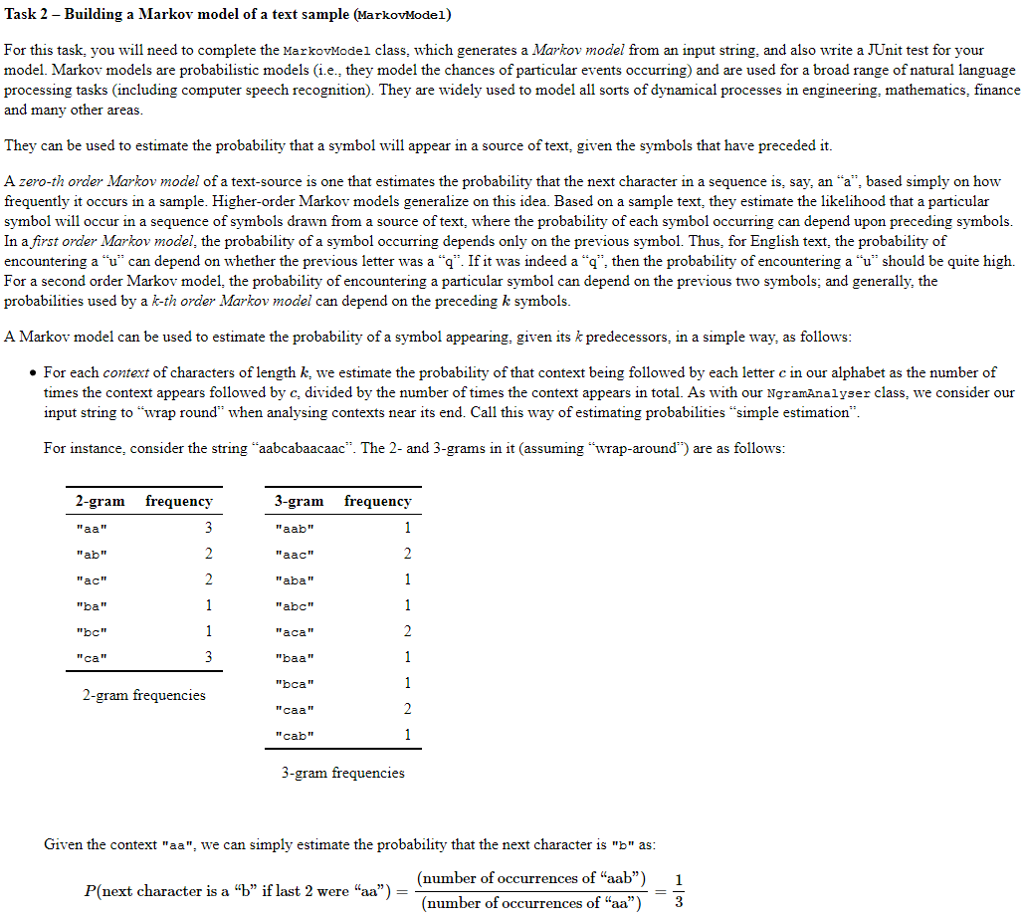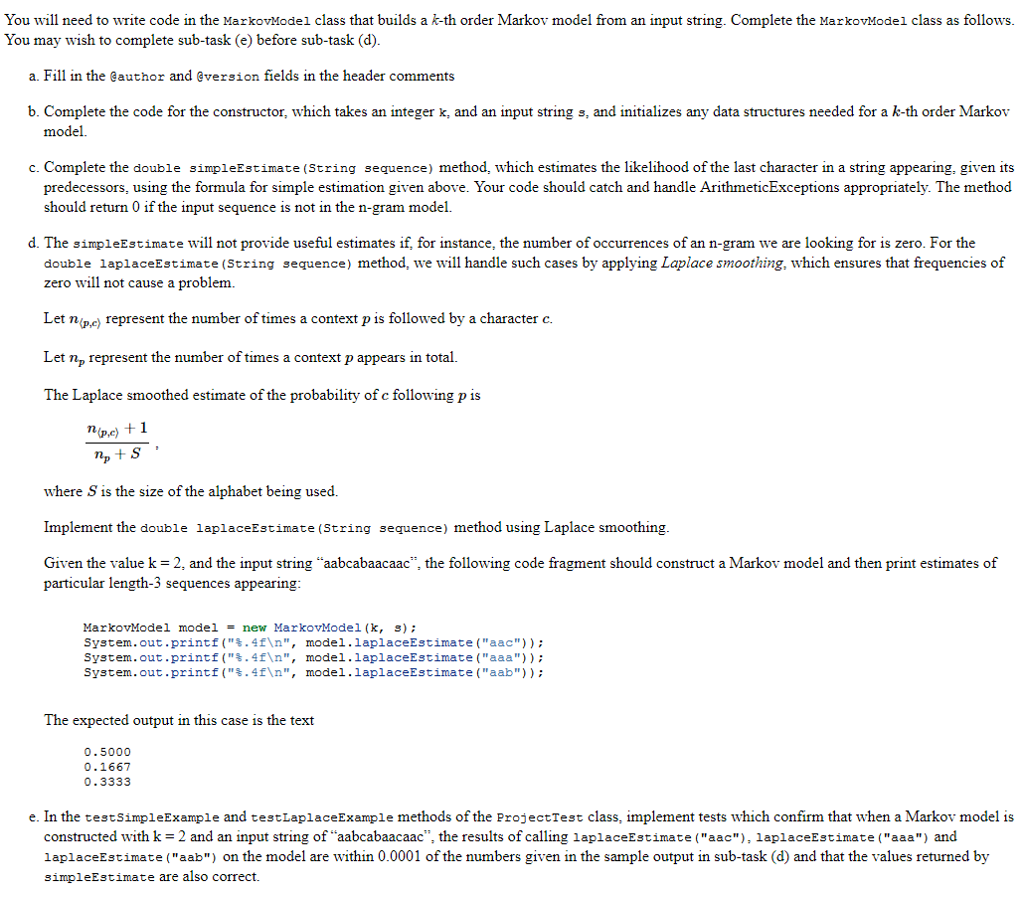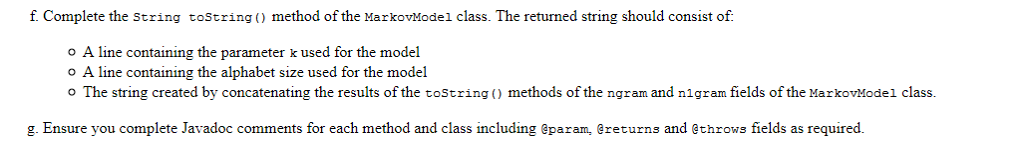<<MarkovModel.java>>

import java.util.Set;
/**
* Construct a Markov model of order /k/ based on an input string.
*
* @author
* @version
*/
public class MarkovModel
{

/** Markov model order parameter */
int k;
/** ngram model of order k */
NgramAnalyser ngram;
/** ngram model of order k+1 */
NgramAnalyser n1gram;

/**
* Construct an order-k Markov model from string s
* @param k int order of the Markov model
* @param s String input to be modelled
*/
public MarkovModel(int k, String s)
{
//TODO replace this line with your code
}

/**
* @return order of this Markov model
*/
public int getK()
{
return k;
}

/** Estimate the probability of a sequence appearing in the text
* using simple estimate of freq seq / frequency front(seq).
* @param sequence String of length k+1
* @return double probability of the last letter occuring in the
* context of the first ones or 0 if front(seq) does not occur.
*/
public double simpleEstimate(String sequence) {
//TODO replace this line with your code
return -1.0;

}
/**
* Calculate the Laplacian probability of string obs given this Markov model
* @input sequence String of length k+1
*/
public double laplaceEstimate(String sequence)
{
//TODO replace this line with your code
return -1.0;
}

/**
* @return String representing this Markov model
*/
public String toString()
{
//TODO replace this line with your code
return null;
}

}

————————————————————————————————————————–

————————————————————————————————————————–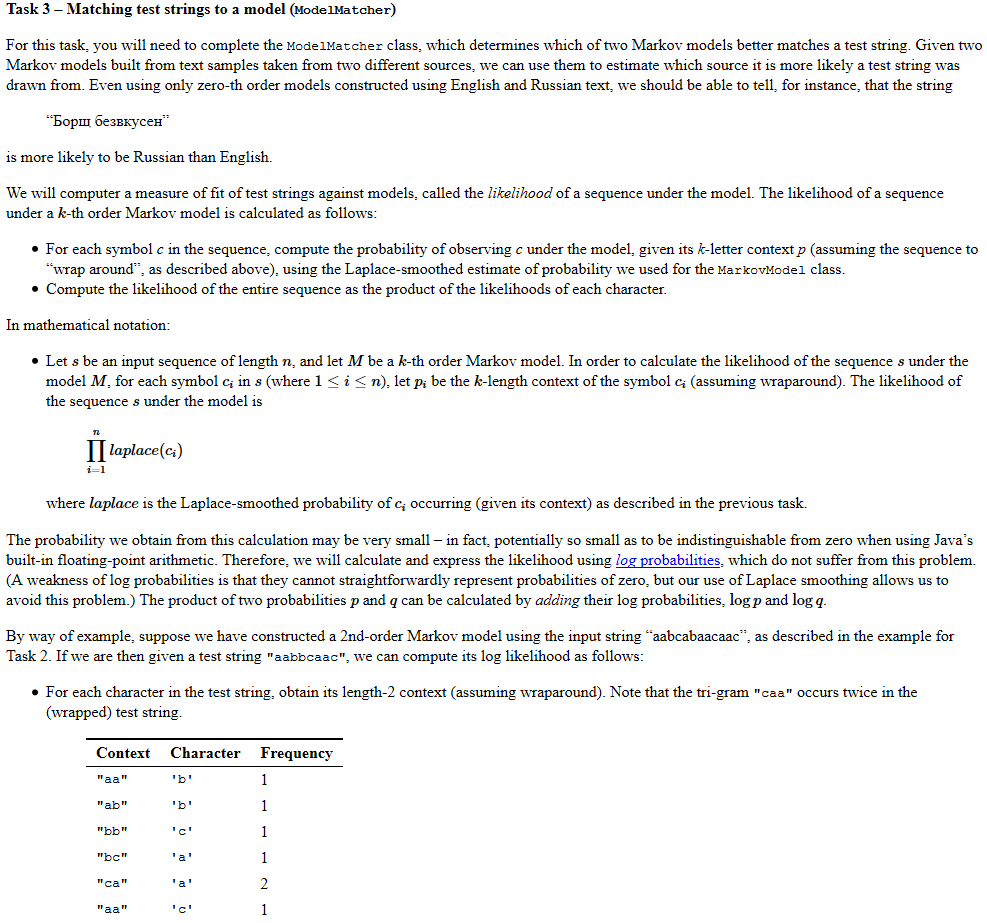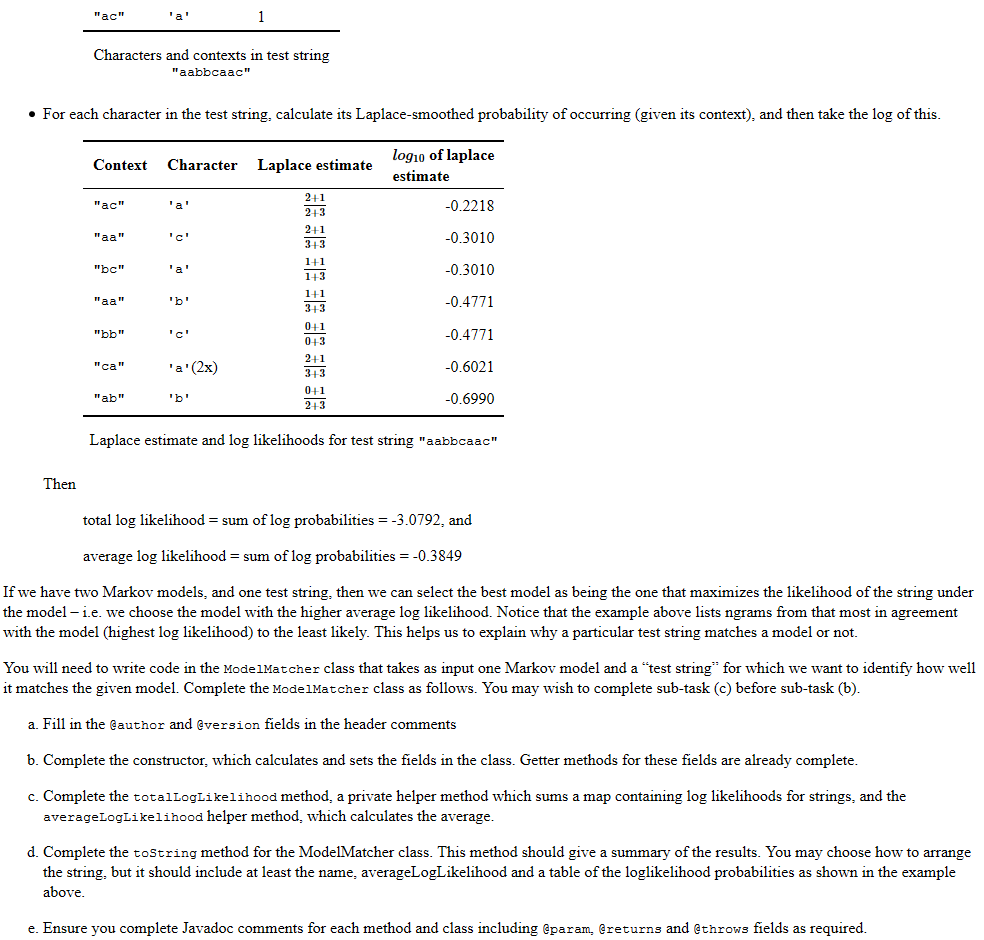<<ModelMatcher.java>>

import java.util.HashMap;
import java.util.Collection;
import java.util.ArrayList;
import java.util.Arrays;

/**
* Report the average log likelihood of a test String occuring in a
* given Markov model and detail the calculated values behind this statistic.
*
* @author
* @version
*/
public class ModelMatcher
{

/** log likelihoods for a teststring under a given model */
private HashMap<String,Double> logLikelihoodMap;
/** summary statistic for this setting */
private double averageLogLikelihood;

/**
* Constructor to initialise the fields for the log likelihood map for
* a test string and a given Markov model and
* the average log likelihood summary statistic
* @param MarkovModel model a given Markov model object
* @param String teststring
*/
public ModelMatcher(MarkovModel model, String testString)
{
//TODO
}

/** Helper method that calculates the average log likelihood statistic
* given a HashMap of strings and their Laplace probabilities
* and the total number of ngrams in the model.
*
* @param logs map of ngram strings and their log likelihood
* @param ngramCount int number of ngrams in the original test string
* @return average log likelihood: the total of loglikelihoods
*    divided by the ngramCount
*/
private double averageLogLikelihood(HashMap<String,Double> logs, int ngramCount)
{
//TODO
return 0.1;
}

/** Helper method to calculate the total log likelihood statistic
* given a HashMap of strings and their Laplace probabilities
* and the total number of ngrams in the model.
*
* @param logs map of ngram strings and their log likelihood
*/
private double totalLogLikelihood(HashMap<String,Double> logs)
{
//TODO
return 0.1;
}

/**
* @return the average log likelihood statistic
*/
public double getAverageLogLikelihood()
{
return averageLogLikelihood;
}

/**
* @return the log likelihood value for a given ngram from the input string
*/
public double getLogLikelihood(String ngram)
{
return (logLikelihoodMap.get(ngram));
}

/**
* Make a String summarising the log likelihood map and its statistics
* @return String of ngrams and their loglikeihood differences between the models
* The likelihood table should be ordered from highest to lowest likelihood
*/
public String toString()
{
//TODO
return null;
}

}

————————————————————————————————————————–

————————————————————————————————————————–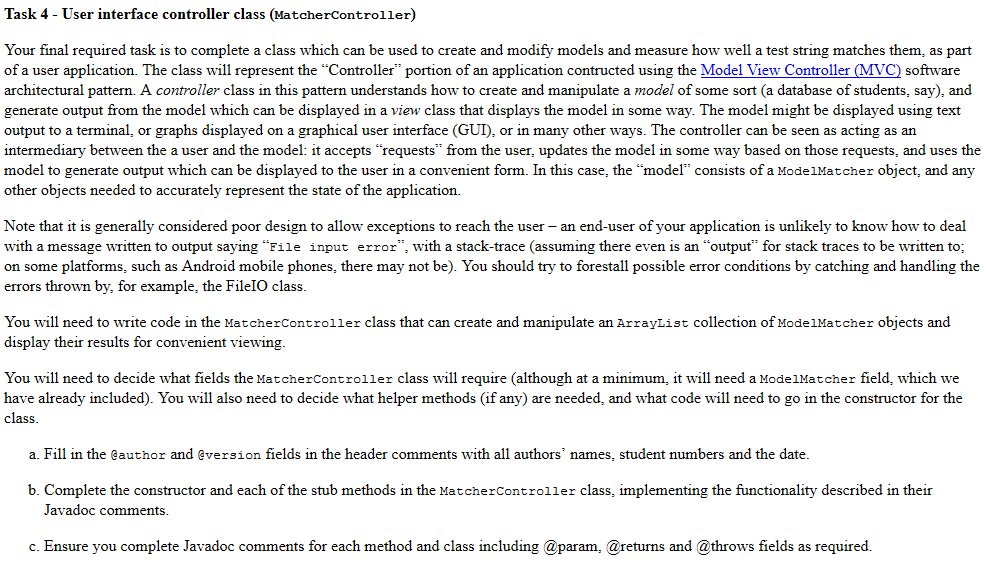<<MatcherController.java>>

import java.io.File;
import java.util.ArrayList;
import java.util.HashMap;
import java.util.Set;
import java.io.*;

/** Create and manipulate Markov models and model matchers for lists of training data
* a test data String and generate output from it for convenient display.
*
* @author
* @version
*
*/
public class MatcherController {

/** list of training data string used to generate markov models */
ArrayList<String> trainingDataList;
/** test data to be matched with the models */
String testData;
/** order of the markov models*/
int k;
/** generated list of markov models for the given training data*/
ArrayList<MarkovModel> modelList;
/** generated list of matchers for the given markov models and test data*/
ArrayList<ModelMatcher> matcherList;

/** Generate models for analysis
* @param k order of the markov models to be used
* @param testData String to check against different models
* @throw unchecked exceptions if the input order or data inputs are invalid
*/
public MatcherController(int k, ArrayList<String> trainingDataList, String testData)
{
//TODO
}

/** @return a string containing all lines from a file
* ff file contents can be got, otherwise null
* This method should process any exceptions that arise.
*/
private static String getFileContents(String filename) {
//TODO
return null;
}

/**
* @return the ModelMatcher object that has the highest average loglikelihood
* (where all candidates are trained for the same test string
*/
public ModelMatcher getBestMatch(ArrayList<ModelMatcher> candidates)
{
//TODO
return null;
}

/** @return String an *explanation* of
* why the test string is the match from the candidate models
*/
public String explainBestMatch(ModelMatcher best) {
//TODO
return null;
}

/** Display an error to the user in a manner appropriate
* for the interface being used.
*
* @param message
*/
public void displayError(String message) {
// LEAVE THIS METHOD EMPTY
}

}

————————————————————————————————————————–

————————————————————————————————————————–

<<ProjectTest.java>>

import static org.junit.Assert.*;
import org.junit.After;
import org.junit.Before;
import org.junit.Test;

/**
* The test class ProjectTest for student test cases.
*
* @author
* @version
*/
public class ProjectTest
{
/**
* Default constructor for test class ProjectTest
*/
public ProjectTest()
{
}

/**
* Sets up the test fixture.
*
* Called before every test case method.
*/
@Before
public void setUp()
{
}

/**
* Tears down the test fixture.
*
* Called after every test case method.
*/
@After
public void tearDown()
{
}

//TODO add new test cases from here include brief documentation

@Test(timeout=1000)
public void testLaplaceExample() {
assertEquals(0,1); //TODO replace with test code
}

@Test(timeout=1000)
public void testSimpleExample() {
assertEquals(0,1); //TODO replace with test code
}

@Test
{
MarkovModel model = new MarkovModel(2,”aabcabaacaac”);
ModelMatcher match = new ModelMatcher(model,”aabbcaac”);
assertEquals(0,1); //TODO replace with test code
}
}

//MarkovModel.java

=========================================================================
import java.util.Set;
/**
* Construct a Markov model of order /k/ based on an input string.
*
*/
public class MarkovModel
{

/** Markov model order parameter */
int k;
/** ngram model of order k */
NgramAnalyser ngram;
/** ngram model of order k+1 */
NgramAnalyser n1gram;
// probability of the next ngram occuring
double probability;
//the size of the alphabet
int alphSize;

/**
* Construct an order-k Markov model from string s
* @param k int order of the Markov model
* @param s String input to be modelled
*/
public MarkovModel(int k, String s)
{
this.k = k;
ngram = new NgramAnalyser (k, s);
n1gram = new NgramAnalyser (k+1, s);
}

/**
* @return order of this Markov model
*/
public int getK()
{
return this.k;
}

/**
* Estimate the probability of a sequence appearing in the text
* using simple estimate of freq seq / frequency front(seq).
* @param sequence String of length k+1
* @return double probability of the last letter occuring in the
* context of the first ones or 0 if front(seq) does not occur.
*/
public double simpleEstimate(String sequence) {
int sequenceFreq = n1gram.getNgramFrequency(sequence);
int preFreq = ngram.getNgramFrequency(sequence.substring(0,sequence.length()-1));
if (sequenceFreq == 0){
probability = 0;
}        else{
probability = (double) sequenceFreq / (double) preFreq;
}
return probability;
}
/**
* Calculate the Laplacian probability of string obs given this Markov model
* @input sequence String of length k+1
*/
public double laplaceEstimate(String sequence)
{
int sequenceFreq = n1gram.getNgramFrequency(sequence);
int preFreq = ngram.getNgramFrequency(sequence.substring(0,sequence.length()-1));
alphSize = ngram.getAlphabetSize();
probability = ((double) (sequenceFreq + 1)) / ((double) (preFreq + alphSize));
return probability;
}

/**
* @return String representing this Markov model
*/
public String toString()
{
String result = “The ” + k + ” order of the Markov Modeln” + “alphabet size of ” + alphSize + “n”;
result += ngram.toString() + n1gram.toString();
System.out.println(result);
return result;
}

}

============================================================================================================
//MatcherController.java
import java.io.File;
import java.util.ArrayList;
import java.util.HashMap;
import java.util.Set;
import java.io.*;

/**
* Create and manipulate Markov models and model matchers for lists of training data
* a test data String and generate output from it for convenient display.
*
*
*/
public class MatcherController {

/** list of training data string used to generate markov models */
ArrayList<String> trainingDataList;
/** test data to be matched with the models */
String testData;
/** order of the markov models */
int k;
/** generated list of markov models for the given training data */
ArrayList<MarkovModel> modelList;
/** generated list of matchers for the given markov models and test data */
ArrayList<ModelMatcher> matcherList;
/** best ModelMatcher for the given testData string */
ModelMatcher bestModelMatcher;

/**
* Generate models for analysis
* Initialize class fields
* @param k order of the markov models to be used
* @param testData String to check against different models
* @throw unchecked exceptions if the input order or data inputs are invalid
*/
public MatcherController(int k, ArrayList<String> trainingDataList, String testData) {

this.checkInputs(k, trainingDataList, testData);

this.k = k;
this.testData = testData;
this.trainingDataList = trainingDataList;
this.modelList = new ArrayList<MarkovModel>();
this.matcherList = new ArrayList<ModelMatcher>();

ModelMatcher tempMatcher;
MarkovModel tempModel;

for (String trainingString : this.trainingDataList) {
tempModel = new MarkovModel(k, trainingString);
tempMatcher = new ModelMatcher(tempModel, testData);

}

this.bestModelMatcher = this.getBestMatch(this.matcherList);

}

/**
* @return a string containing all lines from a file
* if file contents can be got, otherwise null
* This method should process any exceptions that arise.
*/
private static String getFileContents(String filename) {

try {

String outputString = “”;

for (String fileLine : fileLines) {
outputString = outputString + fileLine;
}

return outputString;

} catch(FileNotFoundException e) {
//TODO
} catch(IOException e) {
//TODO
}

return null;
}

/**
* @return the ModelMatcher object that has the highest average loglikelihood
* (where all candidates are trained for the same test string)
*/
public ModelMatcher getBestMatch(ArrayList<ModelMatcher> candidates) {

double bestLikelihood = 0;
ModelMatcher bestMatcher = candidates.get(0);
double tempLikelihood;

for (ModelMatcher matcher : candidates) {
tempLikelihood = matcher.getAverageLogLikelihood();
if (bestLikelihood == 0 || tempLikelihood > bestLikelihood) {
bestLikelihood = tempLikelihood;
bestMatcher = matcher;
}
}

return bestMatcher;
}

/**
* @return String an *explanation* of
* why the test string is the match from the candidate models
* Prints a bar chart of each loglikelihood relative to the lowest loglikelihood
* Table rows are based on the negative inverse of each loglikelihood
* to produce a proportional and increasing group of values.
* Loglikelihoods are first modified as previously stated (inverse and sign changed)
* These modified bar likelihoods are then converted into rations (barNumbers)
* Also shows numerical values next to each table with best one labelled
*/
public String explainBestMatch(ModelMatcher best) {

ArrayList<Double> modifiedLoglikelihoods = new ArrayList<Double>();
ArrayList<Double> normalLoglikelihoods = new ArrayList<Double>();
ArrayList<Long> barNumbers = new ArrayList<Long>();

Double lowestLikelihood = 0.0;
Double loglikelihood = 0.0;
String outputString = “”;

//Retrieve loglikelihoods from matcher array
for (ModelMatcher matcher : this.matcherList) {

loglikelihood = matcher.getAverageLogLikelihood();
//Modify likelihoods and add them to modified array
loglikelihood = -(1 / loglikelihood);
}

//Find the lowest likelihood to scale bar numbers against
for (Double scaledLikelihood : modifiedLoglikelihoods) {
if (lowestLikelihood == 0.0 || scaledLikelihood < lowestLikelihood) {
lowestLikelihood = scaledLikelihood;
}
}

Double relativeLikelihood;
Long barNumber;

//Get ratio of modified likelihoods to lowest likelihood and add to barNumbers
for (int i = 0; i < modifiedLoglikelihoods.size(); i++) {
relativeLikelihood = modifiedLoglikelihoods.get(i);

barNumber = Math.round(relativeLikelihood / lowestLikelihood);
}

outputString += “Table of Average Likelihoods for each Text Souce:”;
Double actualLikelihood;
Long barScaleValue = 1l;

//Ensure the bar lengths aren’t too large
//barScaleValue scales back each barnumber by a whole factor
Long largestBarLength =
Math.round((-(1/best.getAverageLogLikelihood()))/lowestLikelihood);

while (largestBarLength > 25) {
barScaleValue += 1;
largestBarLength = (long)Math.round(largestBarLength / barScaleValue);
}

//Format and create chart
for (int i = 0; i < barNumbers.size(); i++) {

barNumber = barNumbers.get(i);
actualLikelihood = normalLoglikelihoods.get(i);

outputString += “n”;
outputString += String.format(“%.5g”, actualLikelihood);
if (this.matcherList.get(i) == best) {
outputString += “##|”;
} else {
outputString += ” |”;
}
//Prints out barNumber many ‘-‘ characters for rows of chart.
int numberOfDashes = ((int) (long) barNumber)/((int) (long)barScaleValue);
outputString += new String(new char[numberOfDashes]).replace(“”, “-“);
}

return outputString;
}

/**
* Display an error to the user in a manner appropriate
* for the interface being used.
*
* @param message
*/
public void displayError(String message) {
// LEAVE THIS METHOD EMPTY
}

/**
* Helper function to display the results of the analysis of the
* matchers. Does this textually in a simple, formatted manner
* Designed for use with terminal for experienced programmers
*/
public void displayResults() {
String outputString = “”;
outputString += “Displaying Results for Textual Analysis of String”;
outputString += ” ‘” + this.testData + “‘nn”;
outputString += “Comparing Test String Against”;
outputString += ” ” + this.matcherList.size() + ” “;
outputString += “text sourcesnn”;

outputString += “Analysis determines that the String “;
outputString += “was most likely from the following Text Source:nn”;

int bestIndex = this.matcherList.indexOf(this.bestModelMatcher);
String correctSource = this.trainingDataList.get(bestIndex);
String stylisedSource = “”;
//Stylise the source so it fits correctly for convenient viewing
for (int i = 0; i < correctSource.length(); i += 75) {
stylisedSource += NgramAnalyser.splice(correctSource, i, 75);
stylisedSource += “n”;
}

outputString += stylisedSource;

outputString += “nn — Analysis Details — nn”;
outputString += this.explainBestMatch(this.bestModelMatcher);

System.out.println(outputString);

}

/**
* Helper function to sanitize data inputs
* @throws unchecked exceptions:
* – if k is below or equal to zero
* – if k is higher than the testData string length
* – if the trainingData list is null or empty
* – if the testData string is null or empty
* – if any of the strings in the testData list are empty or null
*/

public void checkInputs(int k, ArrayList<String> trainingDataList, String testData) {
if (k <= 0) {
throw new IllegalArgumentException
(“MatcherController: ngram size cannot be below zero”);
} if (k > testData.length()) {
throw new IllegalArgumentException
(“MatcherController: ngram size cannot be larger than string length”);
} if (trainingDataList.size() == 0) {
throw new IllegalArgumentException
(“MatcherController: list of training strings cannot be empty”);
} if (trainingDataList == null) {
throw new IllegalArgumentException
(“MatcherController: list of training strings cannot be uninitialized”);
} if (testData.length() == 0) {
throw new IllegalArgumentException
(“MatcherController: string to test cannot be empty”);
} if (testData == null) {
throw new IllegalArgumentException
(“MatcherController: string cannot be null”);
}

for (String trainingString : trainingDataList) {
if (trainingString.length() == 0 || trainingString == null) {
throw new IllegalArgumentException
(“MatcherController: trainingDataList cannot have empty/null entries”);
}
}
}

}
====================================================================================================================
//ModelMatcher.java
import java.util.HashMap;
import java.util.Collections;
import java.util.ArrayList;
import java.util.Arrays;
import java.util.Map;
import java.util.Set;

/**
* Report the average log likelihood of a test String occuring in a
* given Markov model and detail the calculated values behind this statistic.
*
*/
public class ModelMatcher
{

/** log likelihoods for a teststring under a given model */
private HashMap<String,Double> logLikelihoodMap;
/** summary statistic for this setting */
private double averageLogLikelihood;

/**
* Constructor to initialise the fields for the log likelihood map for
* a test string and a given Markov model and
* the average log likelihood summary statistic
* @param MarkovModel model a given Markov model object
* @param String teststring
*/
public ModelMatcher(MarkovModel model, String testString) {

int modelOrder = model.getK();
this.logLikelihoodMap = new HashMap<String, Double>();

double laplaceEstimate;
double logLikelihood;
String sequence;

NgramAnalyser stringNgram = new NgramAnalyser(modelOrder + 1,testString);

Set<String> distinctNgrams = stringNgram.getDistinctNgrams();

for (String ngram : distinctNgrams) {
laplaceEstimate = model.laplaceEstimate(ngram);
//Use change of base formula to find log(10) likelihood
logLikelihood = Math.log10(laplaceEstimate);

this.logLikelihoodMap.put(ngram, logLikelihood);
}

this.averageLogLikelihood =
this.averageLogLikelihood(this.logLikelihoodMap, stringNgram.getNgramCount());

}

/** Helper method that calculates the average log likelihood statistic
* given a HashMap of strings and their Laplace probabilities
* and the total number of ngrams in the model.
*
* @param logs map of ngram strings and their log likelihood
* @param ngramCount int number of ngrams in the original test string
* @return average log likelihood: the total of loglikelihoods
*    divided by the ngramCount
*/
private double averageLogLikelihood(HashMap<String,Double> logs, int ngramCount) {

double logSum = this.totalLogLikelihood(logs);

return (logSum /((double)ngramCount));
}

/**
* Helper method to calculate the total log likelihood statistic
* given a HashMap of strings and their Laplace probabilities
* and the total number of ngrams in the model.
*
* @param logs map of ngram strings and their log likelihood
*/
private double totalLogLikelihood(HashMap<String,Double> logs) {
double logSum = 0;

for (Map.Entry<String, Double> entry : logs.entrySet()) {
logSum += entry.getValue();
}

return (logSum);
}

/**
* @return the average log likelihood statistic
*/
public double getAverageLogLikelihood() {
return averageLogLikelihood;
}

/**
* @return the log likelihood value for a given ngram from the input string
*/
public double getLogLikelihood(String ngram) {
return (logLikelihoodMap.get(ngram));
}

/**
* Make a String summarising the log likelihood map and its statistics
* @return Header lines containing the testString, the averageLogLikelihood
*         and a sorted table of ngrams and their loglikelihoods
* The likelihood table should be ordered from highest to lowest likelihood
*/
public String toString() {
String returnString = “”;

returnString = returnString + Double.toString(this.averageLogLikelihood);
returnString = returnString + this.hashMapToString(this.logLikelihoodMap);

return returnString;
}

/**
* Helper function to return a sorted hashmap table string
* Sorted by loglikelihoods from highest to lowest
* @param the map to be sorted and printed
* @return the string of the hashmap table
*/

private String hashMapToString(HashMap<String, Double> map) {

HashMap<Double, String> reversedMap = new HashMap<Double, String>();
ArrayList<Double> likelihoods = new ArrayList<Double>();

for (Map.Entry<String, Double> entry : map.entrySet()) {
reversedMap.put(entry.getValue(), entry.getKey());
}

Collections.sort(likelihoods);
String outputString = “”;

for (Double likelihood : likelihoods) {
outputString += reversedMap.get(likelihood);
outputString += ” “;
outputString += Double.toString(likelihood);
outputString += “n”;
}

//Remove final n
outputString = NgramAnalyser.splice(outputString, 0, outputString.length() – 1);

return outputString;

}

}
=================================================================================================================
//ProjectTest.java
import static org.junit.Assert.*;
import org.junit.After;
import org.junit.Before;
import org.junit.Test;
import java.util.Set;
import java.util.ArrayList;
import java.util.Arrays;

/**
* The test class ProjectTest for student test cases.
*/
public class ProjectTest
{
/**
* Default constructor for test class ProjectTest
*/
public ProjectTest() {

}

/**
* Sets up the test fixture.
*
* Called before every test case method.
*/
@Before
public void setUp() {
}

/**
* Tears down the test fixture.
*
* Called after every test case method.
*/
@After
public void tearDown() {

}

//TODO add new test cases from here include brief documentation

/**
* Test MarkovModel and all the contained functions
*/

@Test(timeout=1000)
public void testMarkovModel() {

String modelString = “12233321221123231”;
MarkovModel model = new MarkovModel(2, modelString);
//Testing for various sequences
String sequence = “123”;
assert(model.laplaceEstimate(sequence) == (double)(1.0/3.0));
assert(model.simpleEstimate(sequence) == (double)(1.0/3.0));
sequence = “111”;
assert(model.laplaceEstimate(sequence) == (double)(1.0/5.0));
assert(model.simpleEstimate(sequence) == (double)(0.0));
//Test if 0 is returned when given ngram that isnt in string
sequence = “abc”;
assert(model.simpleEstimate(sequence) == (double)(0.0));

}

@Test(timeout=1000)
public void testLaplaceExample() {

String modelString = “aabcabaacaac”;
MarkovModel model = new MarkovModel(2, modelString);
//Testing for various sequences
String[] stringsToTest = {“aac”, “aaa”, “aab”};
double[] correctLaplace = {(1.0/2.0), (1.0/6.0), (1.0/3.0)};

for (int i = 0; i < 3; i++) {
assert(model.laplaceEstimate(stringsToTest[i]) == correctLaplace[i]);
}
}

@Test(timeout=1000)
public void testSimpleExample() {
String modelString = “aabcabaacaac”;
MarkovModel model = new MarkovModel(2, modelString);
//Testing for various sequences
String[] stringsToTest = {“aac”, “aaa”, “aab”};
double[] correctSimple = {(2.0/3.0), (0.0), (1.0/3.0)};

for (int i = 0; i < 3; i++) {
assert(model.simpleEstimate(stringsToTest[i]) == correctSimple[i]);
}

}

@Test
/*
In this case the absolute value of the output had to be taken. This is acceptable because the output will never produce a positive number.
The boundary case would be an ngram which only contains a single character type, like “aaaaa”, then the alphabet size will be 1 and if
looking for “aaa”, the laplace estimation will be 1 and the log will be 0. Any other case will result in a laplace estimate being a
fraction < 1, resulting in a negative log value.
*/
MarkovModel model = new MarkovModel(2,”aabcabaacaac”);
ModelMatcher match = new ModelMatcher(model,”aabbcaac”);
assertEquals((int)Math.abs(Math.round(match.getAverageLogLikelihood()*10000)) ,3849);
}
}Order NOW for a 10% Discount
Pages (550 words)
Approximate price: -

Why Us?Top Quality and Well-Researched Papers

All ourbpapers are written from scratch. In fact, Clients who ask for paraphrasing services are highly discouraged. We have writers ready to craft any paper from scratch and deliver quality ahead of time.Our writers keeps you posted on your papers progress - providing you with paper outline/draft. You are also at liberty to communicate directly with your writer.Free Unlimited Revisions

If you think we missed something, send your order for a free revision. You have 10 days to submit the order for review after you have received the final document. You can do this yourself after logging into your personal account or by contacting our support.Prompt Delivery and 100% Money-Back-Guarantee

All papers are always delivered on time, in many cases quite ahead of time. In case we need more time to master your paper, we may contact you regarding the deadline extension. In case you cannot provide us with more time, a 100% refund is guaranteed.Original & Confidential

We use several writing tools checks to ensure that all documents you receive are free from plagiarism. Our editors carefully review all quotations in the text. We also promise maximum confidentiality in all of our services.Our support agents are available 24 hours a day 7 days a week and committed to providing you with the best customer experience. Get in touch whenever you need any assistance.

Try it now!

## Calculate the price of your order

Total price:
\$0.00

How it works?Fill in the order form and provide all details of your assignment.Proceed with the payment

Choose the payment system that suits you most.Our Services

No need to work on your paper at night. Sleep tight, we will cover your back. We offer all kinds of writing services.## Essay Writing Service

No matter what kind of academic paper you need and how urgent you need it, you are welcome to choose your academic level and the type of your paper at an affordable price. We take care of all your paper needs and give a 24/7 customer care support system.An admission essay is an essay or other written statement by a candidate, often a potential student enrolling in a college, university, or graduate school. You can be rest assurred that through our service we will write the best admission essay for you.Editing Support

Our academic writers and editors make the necessary changes to your paper so that it is polished. We also format your document by correctly quoting the sources and creating reference lists in the formats APA, Harvard, MLA, Chicago / Turabian.Revision Support

If you think your paper could be improved, you can request a review. In this case, your paper will be checked by the writer or assigned to an editor. You can use this option as many times as you see fit. This is free because we want you to be completely satisfied with the service offered.